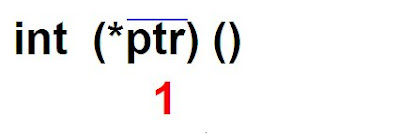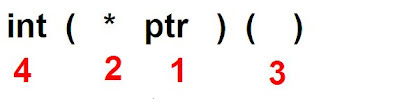## INDEX

### How will we read complex pointer ?

Rule1. Give the first priority to the name (identifier) of pointer variable.

Rule2. Give the least priority of to the data type with modifier (like int, char, float, unsigned int, static int etc.)

Rule3. () and [] operator has higher priority (associatively left to right) than *, & (associatively right to left)

Priority: It means operator which has highest priority will execute first.

Associatively: If associatively is left to right and two operator have same priority then left most operator will have more priority and vice versa.

If right side of name (identifier) is ( ) then read function after the function read all as return type
* pointer if you have not encounter function or array otherwise read address. If you will read example then you will understand more easily.

Example1:
int (*ptr)();
Answer:Give first priority to ptr :1There are two () operator ,associatively of () operator is left to right so left most operator will have more priority.Left most ( ) operator will execute first. So give * to the second priority and right most ( ) operator to the third priority.
Give fourth priority to intRead: ptr is pointer to such function which return type is integer data type.

#include<stdio.h>
int main(){
int (*ptr1)();
int (*ptr2)();
void (*ptr3)();
ptr1=puts;
ptr2=getch;
ptr3=clrscr;
(*ptr3)();
(*ptr1)("POWER OF POINTER");
(*ptr2)();
return 0;
}

Output: POWER OF POINTER

Example 2.
char *arr ;
arr is array of size 3 which contain address of char
program:
#include<stdio.h>
int main(){
char *arr;
char a=1,b=2,c=3;
arr=&a;
arr=&b;
arr=&c;
printf("%u %u",&b,arr);
printf("\n%d",*arr);
return 0;
}
Output :
3

Example 3
char (*ptr);

ptr is pointer to array of size 5 which contain char data type.

Program:
#include<stdio.h>
int main(){
char arr={1,2,3,4,5};
char (*ptr);
ptr=&arr;
ptr=(*ptr)+2;
printf("%d",**ptr);
return 0;
}
Output: 3
Example 4
unsigned long int ( * avg ())[ 3]

avg is such function which return type is address of array of size of 3 which contain unsigned long int data type.

Program:
#include<stdio.h>
unsigned long int (* avg()){
static unsigned long int arr={1,2,3};
return &arr;
}
int main(){
unsigned long int (*ptr);
ptr=avg();
printf("%d",*(*ptr+2));
return 0;
}
Output :3

#### 1 comment:

NICKS said...

good questions...some more will really be appreciated